# 1 Performing variable selection

• Forward selection, backward elimination, and branch and bound selection can be done using VariableSelection().
• VariableSelection() can accept either a BranchGLM object or a formula along with the data and the desired family and link to perform the variable selection.
• Available metrics are AIC, BIC and HQIC, which are used to compare models and to select the best models.
• VariableSelection() returns some information about the search, more detailed information about the best models can be seen by using the summary() function.
• Note that VariableSelection() will properly handle interaction terms and categorical variables.
• keep can also be specified if any set of variables are desired to be kept in every model.

## 1.1 Metrics

• The 3 different metrics available for comparing models are the following
• Akaike information criterion (AIC), which typically results in models that are useful for prediction
• $$AIC = -2logLik + 2 \times p$$
• Bayesian information criterion (BIC), which results in models that are more parsimonious than those selected by AIC
• $$BIC = -2logLik + \log{(n)} \times p$$
• Hannan-Quinn information criterion (HQIC), which is in the middle of AIC and BIC
• $$HQIC = -2logLik + 2 * \log({\log{(n)})} \times p$$

## 1.2 Stepwise methods

• Forward selection and backward elimination are both stepwise variable selection methods.
• They are not guaranteed to find the best model or even a good model, but they are very fast.
• Forward selection is recommended if the number of variables is greater than the number of observations or if many of the larger models don’t converge.
• These methods will only return 1 best model.
• Parallel computation can be used for the methods, but is generally only necessary for large datasets.

### 1.2.1 Forward selection example

# Loading BranchGLM package
library(BranchGLM)

# Fitting gamma regression model
cars <- mtcars

# Fitting gamma regression with inverse link
GammaFit <- BranchGLM(mpg ~ ., data = cars, family = "gamma", link = "inverse")

# Forward selection with mtcars
forwardVS <- VariableSelection(GammaFit, type = "forward")
forwardVS
#> Variable Selection Info:
#> ------------------------
#> Variables were selected using forward selection with AIC
#> The best value of AIC obtained was 142.2
#> Number of models fit: 27
#> Variables that were kept in each model:  (Intercept)
#> Order the variables were added to the model:
#>
#> 1). wt
#> 2). hp

## Getting final model
fit(forwardVS, which = 1)
#> Results from gamma regression with inverse link function
#> Using the formula mpg ~ hp + wt
#>
#>              Estimate SE  t p.values
#> (Intercept) 8.923e-03 NA NA       NA
#> hp          8.887e-05 NA NA       NA
#> wt          9.826e-03 NA NA       NA
#>
#> Dispersion parameter taken to be 0.0104
#> 32 observations used to fit model
#> (0 observations removed due to missingness)
#>
#> Residual Deviance: 0.33 on 29 degrees of freedom
#> AIC: 142.2
#> Algorithm converged in 3 iterations using Fisher's scoring

### 1.2.2 Backward elimination example

# Backward elimination with mtcars
backwardVS <- VariableSelection(GammaFit, type = "backward")
backwardVS
#> Variable Selection Info:
#> ------------------------
#> Variables were selected using backward elimination with AIC
#> The best value of AIC obtained was 141.9
#> Number of models fit: 49
#> Variables that were kept in each model:  (Intercept)
#> Order the variables were removed from the model:
#>
#> 1). vs
#> 2). drat
#> 3). am
#> 4). disp
#> 5). carb
#> 6). cyl

## Getting final model
fit(backwardVS, which = 1)
#> Results from gamma regression with inverse link function
#> Using the formula mpg ~ hp + wt + qsec + gear
#>
#>               Estimate SE  t p.values
#> (Intercept)  4.691e-02 NA NA       NA
#> hp           6.284e-05 NA NA       NA
#> wt           9.485e-03 NA NA       NA
#> qsec        -1.299e-03 NA NA       NA
#> gear        -2.662e-03 NA NA       NA
#>
#> Dispersion parameter taken to be 0.0091
#> 32 observations used to fit model
#> (0 observations removed due to missingness)
#>
#> Residual Deviance: 0.29 on 27 degrees of freedom
#> AIC: 141.9
#> Algorithm converged in 3 iterations using Fisher's scoring

## 1.3 Branch and bound

• The branch and bound methods can be much slower than the stepwise methods, but they are guaranteed to find the best models.
• The branch and bound methods are typically much faster than an exhaustive search and can also be made even faster if parallel computation is used.

### 1.3.1 Branch and bound example

• If showprogress is true, then progress of the branch and bound algorithm will be reported occasionally.
• Parallel computation can be used with these methods and can lead to very large speedups.
# Branch and bound with mtcars
VS <- VariableSelection(GammaFit, type = "branch and bound", showprogress = FALSE)
VS
#> Variable Selection Info:
#> ------------------------
#> Variables were selected using branch and bound selection with AIC
#> The best value of AIC obtained was 141.9
#> Number of models fit: 63
#> Variables that were kept in each model:  (Intercept)

## Getting final model
fit(VS, which = 1)
#> Results from gamma regression with inverse link function
#> Using the formula mpg ~ hp + wt + qsec + gear
#>
#>               Estimate SE  t p.values
#> (Intercept)  4.691e-02 NA NA       NA
#> hp           6.284e-05 NA NA       NA
#> wt           9.485e-03 NA NA       NA
#> qsec        -1.299e-03 NA NA       NA
#> gear        -2.662e-03 NA NA       NA
#>
#> Dispersion parameter taken to be 0.0091
#> 32 observations used to fit model
#> (0 observations removed due to missingness)
#>
#> Residual Deviance: 0.29 on 27 degrees of freedom
#> AIC: 141.9
#> Algorithm converged in 3 iterations using Fisher's scoring
• A formula with the data and the necessary BranchGLM fitting information can also be used instead of supplying a BranchGLM object.
# Can also use a formula and data
formulaVS <- VariableSelection(mpg ~ . ,data = cars, family = "gamma",
link = "inverse", type = "branch and bound",
showprogress = FALSE, metric = "AIC")
formulaVS
#> Variable Selection Info:
#> ------------------------
#> Variables were selected using branch and bound selection with AIC
#> The best value of AIC obtained was 141.9
#> Number of models fit: 63
#> Variables that were kept in each model:  (Intercept)

## Getting final model
fit(formulaVS, which = 1)
#> Results from gamma regression with inverse link function
#> Using the formula mpg ~ hp + wt + qsec + gear
#>
#>               Estimate SE  t p.values
#> (Intercept)  4.691e-02 NA NA       NA
#> hp           6.284e-05 NA NA       NA
#> wt           9.485e-03 NA NA       NA
#> qsec        -1.299e-03 NA NA       NA
#> gear        -2.662e-03 NA NA       NA
#>
#> Dispersion parameter taken to be 0.0091
#> 32 observations used to fit model
#> (0 observations removed due to missingness)
#>
#> Residual Deviance: 0.29 on 27 degrees of freedom
#> AIC: 141.9
#> Algorithm converged in 3 iterations using Fisher's scoring

### 1.3.2 Using bestmodels

• The bestmodels argument can be used to find the top k models according to the metric.
# Finding top 10 models
formulaVS <- VariableSelection(mpg ~ . ,data = cars, family = "gamma",
link = "inverse", type = "branch and bound",
showprogress = FALSE, metric = "AIC",
bestmodels = 10)
formulaVS
#> Variable Selection Info:
#> ------------------------
#> Variables were selected using branch and bound selection with AIC
#> Found the top 10 models
#> The range of AIC values for the top 10 models is (141.9, 143.59)
#> Number of models fit: 122
#> Variables that were kept in each model:  (Intercept)

## Plotting results
plot(formulaVS, type = "b")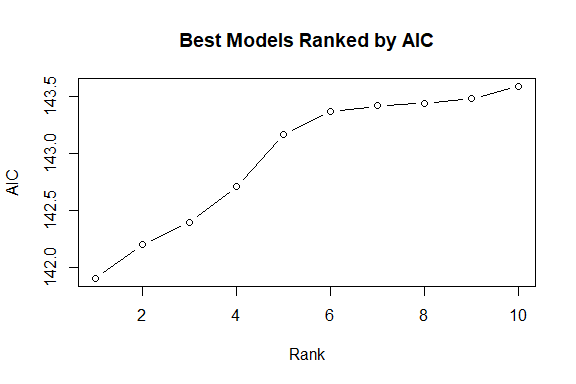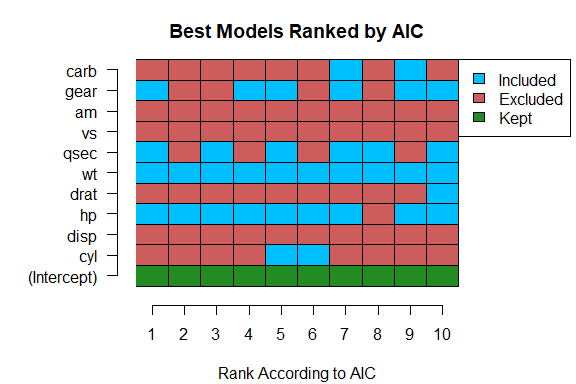## Getting best model
fit(formulaVS, which = 1)
#> Results from gamma regression with inverse link function
#> Using the formula mpg ~ hp + wt + qsec + gear
#>
#>               Estimate SE  t p.values
#> (Intercept)  4.691e-02 NA NA       NA
#> hp           6.284e-05 NA NA       NA
#> wt           9.485e-03 NA NA       NA
#> qsec        -1.299e-03 NA NA       NA
#> gear        -2.662e-03 NA NA       NA
#>
#> Dispersion parameter taken to be 0.0091
#> 32 observations used to fit model
#> (0 observations removed due to missingness)
#>
#> Residual Deviance: 0.29 on 27 degrees of freedom
#> AIC: 141.9
#> Algorithm converged in 3 iterations using Fisher's scoring

### 1.3.3 Using cutoff

• The cutoff argument can be used to find all models that have a metric value that is within cutoff of the minimum metric value found.
# Finding all models with an AIC within 2 of the best model
formulaVS <- VariableSelection(mpg ~ . ,data = cars, family = "gamma",
link = "inverse", type = "branch and bound",
showprogress = FALSE, metric = "AIC",
cutoff = 2)
formulaVS
#> Variable Selection Info:
#> ------------------------
#> Variables were selected using branch and bound selection with AIC
#> Found the top 16 models
#> The range of AIC values for the top 16 models is (141.9, 143.9)
#> Number of models fit: 116
#> Variables that were kept in each model:  (Intercept)

## Plotting results
plot(formulaVS, type = "b")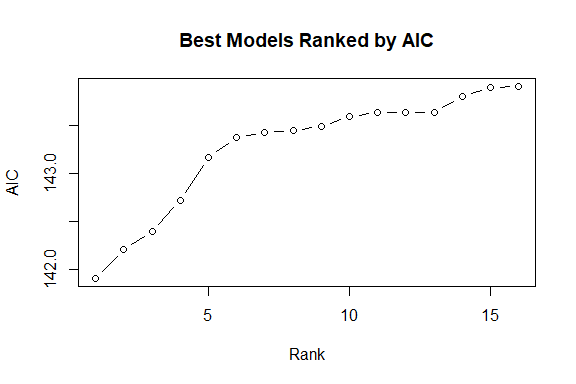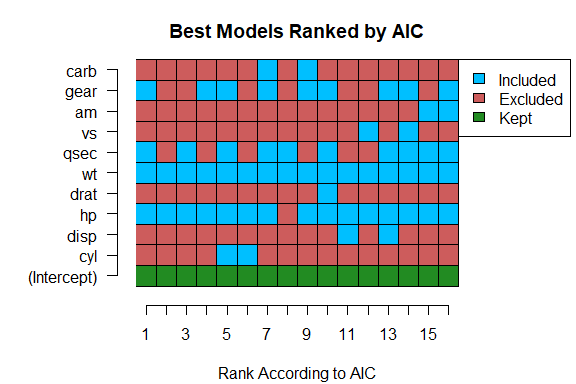## 1.4 Using keep

• Specifying variables via keep will ensure that those variables are kept through the selection process.
# Example of using keep
keepVS <- VariableSelection(mpg ~ . ,data = cars, family = "gamma",
link = "inverse", type = "branch and bound",
keep = c("hp", "cyl"), metric = "AIC",
showprogress = FALSE, bestmodels = 10)
keepVS
#> Variable Selection Info:
#> ------------------------
#> Variables were selected using branch and bound selection with AIC
#> Found the top 10 models
#> The range of AIC values for the top 10 models is (143.17, 145.24)
#> Number of models fit: 55
#> Variables that were kept in each model:  (Intercept), hp, cyl

## Getting summary and plotting results
plot(keepVS, type = "b")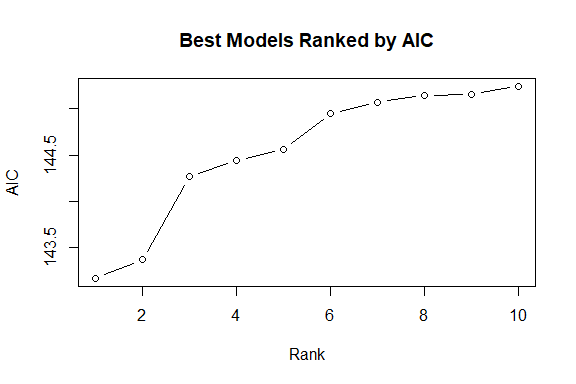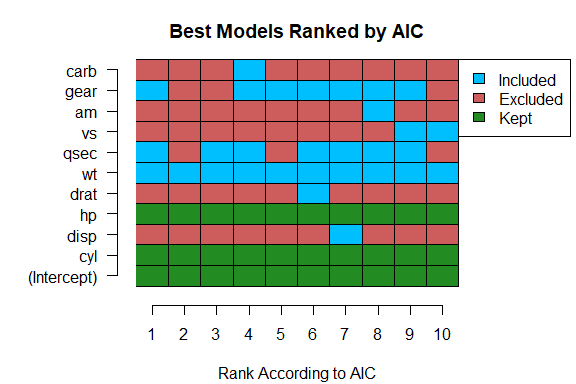## Getting final model
fit(keepVS, which = 1)
#> Results from gamma regression with inverse link function
#> Using the formula mpg ~ cyl + hp + wt + qsec + gear
#>
#>               Estimate SE  t p.values
#> (Intercept)  6.464e-02 NA NA       NA
#> cyl         -1.412e-03 NA NA       NA
#> hp           7.523e-05 NA NA       NA
#> wt           1.037e-02 NA NA       NA
#> qsec        -1.816e-03 NA NA       NA
#> gear        -3.861e-03 NA NA       NA
#>
#> Dispersion parameter taken to be 0.0089
#> 32 observations used to fit model
#> (0 observations removed due to missingness)
#>
#> Residual Deviance: 0.29 on 26 degrees of freedom
#> AIC: 143.17
#> Algorithm converged in 3 iterations using Fisher's scoring

## 1.5 Convergence issues

• It is not recommended to use branch and bound if the upper models do not converge since it can make the algorithm very slow.
• Sometimes when using backwards selection and all the upper models that are tested do not converge, no final model can be selected.
• For these reasons, if there are convergence issues it is recommended to use forward selection.